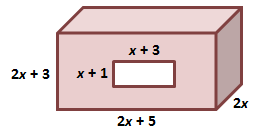#### MAT-HS.A-APR.01

 MAT-HS Targeted Standards(A) Concept: Algebra(SSE) Domain: Arithmetic with Polynomials and Rational ExpressionsCluster: Perform arithmetic operations on polynomials MAT-HS.A-APR.01 Add, subtract, and multiply polynomials. Understand that polynomials form a system analogous to the integers, namely, they are closed under the operations of addition, subtraction, and multiplication.

## Student Learning Targets:

### Knowledge Targets

• I can identify polynomials.

### Reasoning Targets

• I can recognize how closure applies under these operations.
• I can

### Skills (Performance) Targets

• I can add, subtract, and multiply polynomials and recognize how closure applies under these operations.

• I can
• I can

## Proficiency Scale

 Score Description Sample Activity 4.0 (advanced) In addition to Score 3.0, the student demonstrates in-depth inferences and applications regarding more complex material that go beyond end of instruction expectations. Shannon, a cabinetmaker, started out with a block of wood, and then she hollowed out the center of the block.  The dimensions of the block and the cutout is shown below. Write the volume of the original block, and the volume of the hole. (ignore units for this problem) Write the polynomial for the volume of the wood remaining.3.5 In addition to Score 3.0 performance, the student demonstrates in-depth inferences and applications regarding the more complex content with partial success. 3.0 (proficient) The student can: add, subtract, and multiply polynomials. recognize that when two polynomials are added, subtracted, or multiplied, a polynomial is the result. The student exhibits no major errors or omissions. Simplify the following: (2x2+3x+5)+(5x3-9x-8) (x4+3x3-x2)-(4x3+8) (x+2)(5x2-7x+10) 2.5 The student demonstrates no major errors or omissions regarding the simpler details and processes (Score 2.0 content) and partial knowledge of the more complex ideas and processes (Score 3.0 content). 2.0 (progressing) There are no major errors or omissions regarding the simpler details and processes as the student can: recognize and recall specific terminology, such as: polynomial trinomial binomial term combine like terms. However, the student exhibits major errors or omissions regarding the more complex ideas and processes. Simplify the following: 2x2+7x-9+3x2+5x-10 (2x+3)(4x-8) 1.5 The student demonstrates partial knowledge of the simpler details and processes (Score 2.0 content) but exhibits major errors or omissions regarding the more complex ideas and procedures (Score 3.0 content). 1.0 (beginning) With help, the student demonstrates a partial understanding of some of the simpler details and processes (Score 2.0 content) and some of the more complex ideas and processes (Score 3.0 content). 0.5 With help, the student demonstrates a partial understanding of some of the simpler details and processes (Score 2.0 content) but not the more complex ideas and processes (Score 3.0 content). 0.0 Even with help, the student demonstrates no understanding or skill.

## Resources

 Web Vocab Polynomial